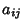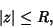## Perron-Frobenius Theorem

If all elementsof an Irreducible Matrixare Nonnegative, thenis an Eigenvalue ofand all the Eigenvalues oflie on the Diskwhere, ifis a set of Nonnegative numbers (which are not all zero),and. Furthermore, ifhas exactlyEigenvalueson the Circle, then the set of all its Eigenvalues is invariant under rotations byabout the Origin.